# The Geometric Viewpoint

geometric and topological excursions by and for undergraduates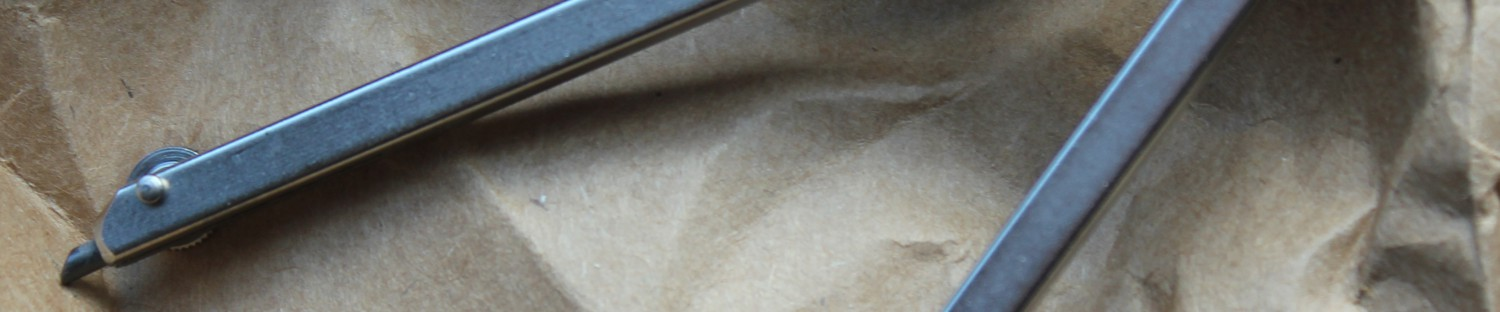## Using Configuration Spaces to Find Inscribed Squares

As an undergraduate in mathematics, every once in a while, one of your math friends will send you a link to a very interesting unsolved problem in mathematics.  I made the mistake of clicking on one, because after reading it, it captivated my mind for weeks: “The Inscribed Square Problem.” Now, this conjecture says that for any simple closed curve (Jordan curve), there is a square whose corner points all lie in the curve. On first examination, I thought to myself, “wow there’s no way this is true, there are some pretty messed up curves out there, and I bet there’s at least one of them where this does not hold.” So I went to talk to my topology professor about this and we talked about a method of approaching this problem using configuration spaces, which I will talk a little bit about in this blog post.

First we will start off by explaining exactly what a configuration space is. A configuration space is a way to topologically represent how objects can be placed and moved around within certain spaces. For example, if you have a circle and you want to place two points on it, you might start by thinking that for every place you put your first point, the places that you could place the second point can be represented by a circle with a point removed (because both points cannot be in the same place), and so it turns out that the configuration space of two points in a circle (denoted$C^2(S^1)$ where$S^1$ is the circle and the superscript 2 denotes how many bodies are in the space) is$S^1 \times(S^1 - \{a\})$ where a is a point on the circle. Now this is interesting, because$S^1 \times S^1$ is an interesting construction called the torus (it’s pretty much just a doughnut):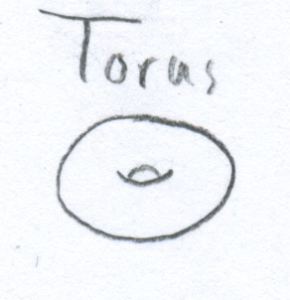However, removing a point from the second circle transforms it into a line segmentso you end up with something similar to$S^1 \times (S^1 - \{a\})$ which looks like the result of rolling a piece of paper up. (Something akin to the outside shell of a hollow cylinder without the circles on the ends)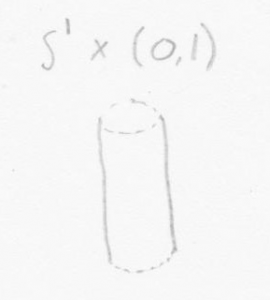Now, while you may believe me when I say that each point on this gives a place for two positions on the circle, let us examine how this actually works, here I have drawn a circle with two points A and B on it (I have also drawn an arrow at the top as a reference point).  First let us choose our A position.  The place around the cylinder determines where our first point should go.  We want to place our A point somewhere on the cylinder where the dashed-and-dotted line is because that is where A is in relation to our point of reference (note that if we were to look at the cylinder from the top, that line would correspond exactly to where A is).  Now, the height at which we place our B point on the cylinder is determined by where in relation to A it is.  Here we can see that B is a little after A if we head clockwise around the circle.  So we can place B a little bit away from the top to get the point shown on our cylinder.  Note that this also demonstrates why the bottom and top of our cylinders are open, because as we get to the edges of the cylinder, we get closer and closer to the A point, but can’t actually reach it.  If we could, we could say that the top and bottom of our cylinder were essentially the same circle and “glue” them together to get the torus.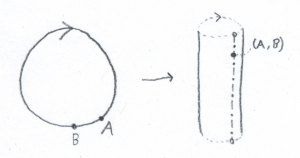Now, a Jordan curve in the plane can be described as the image of an injective continuous function from the unit circle to the plane. Knowing that our goal is to try and determine whether it has an inscribed square, we should start by examining how 4 points can be configured on our curve, that is$C^4(\phi(S^1))$ where$\phi$ is our injective continuous function. Now, because our curve is continuous and does not cross over itself, it is essentially just a circle which has been squished and pulled to make it our circle, so by reversing our process (finding a continuous function which when composed with$\phi$ gives us a circle), we can deform it back into the circle: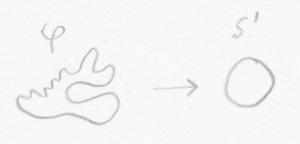and by doing this, we can notice a few things about the structure of our configuration space. Firstly we already know how to place 2 points on the circle, but we can also realize that placing four points on the circle is exactly the same as placing 2 points on the circle twice! (This is fairly obvious in real life, but its mathematical meaning has a bit more significance) that is$C^4(S^1) = C^{2}(C^{2}(S^{1}))$

So, we can look at the configuration space of$C^2(\phi(S^1))$ and notice that it will be equal to$C^{2}(C^{2}(S^{1})) = (C^{2}S^{1}))\times(C^{2}(S^{1})-\{a\})$

So what on earth does this mean? It means that for every point in one of our “cylinders” we can represent two point on the circle by placing one point in our cylinder-like construction and then placing our second point on a different cylinder-like construction with a point missing (Taking the cross product of the two spaces)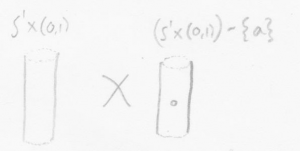Alternatively, you could just declare your configuration space to be$C^{4}(\phi(S^{1})) = \{(a,b,c,d)\in S^{1} | a\ne b\ne c\ne d \}$

But I find the first definition to be much easier to picture in my head than the second succinct but abstract one.
So, now that we have a rough picture of a configuration space for if our curve was a circle, remember that we simplified this by stretching it into a circle, now we can translate it back to our initial curve,$\phi$, by twisting and bending every circle that we encounter back into our Jordan curve (slightly more formally, we have been composing$\phi$ with a function which continuously deforms it into a circle.  At this point, we stop composing$\phi$ with that function).

Now, let us talk briefly about how we can define some fairly useful functions on configuration spaces.  First let’s look at the distance function$d:C^2(S^1)\rightarrow \mathbb{R}$.  First let us not that this function is continuous, if we change our point in the space by a tiny bit, the distance between the two points will not change drastically.  Also we can see that the closer you get to the edges of the cylinder, the closer the two points are (at the edges, they would occupy the same space), so from this, we can see that the maximum distance is the ring in the very center of our cylinder.  This is a very neat way of seeing that the points in the ring in the center of our cylinder occur exactly when our two points on the circle are placed opposite each other.

We can now define very useful function$f$ from$C^4(\phi(S^1))$ to the set {0,1} such that a point$x \in C^4(\phi(S^1))$ is mapped to 1 if one of the components (the points coming from each mutilated circle)$x_1$ is a distance c away from$x_2$ and$x_3$ and is a distance$c\sqrt{2}$ away from the third one, and furthermore, that$x_3,x_1,x_2$ form a right angle and that$x_1,x_2,x_4$ form a right angle. This will ensure that any point in the configuration space that would make a square in the original curve (remember that the configuration space has coordinates for four points on the curve) gets mapped to 1. Now, we can proceed from here in several different ways, we could take the preimage$f^{-1}(1)$ and determine if it is not empty, or we could define the topology on our configuration space to be the indiscrete topology, (the one where the only open sets are the empty set and the entire space), this has the benefit that the only functions which are continuous are constant functions. So, since there will be at least one point in our configuration space which gets mapped to 0 (otherwise every set of four points is an inscribed square, which is obviously not the case), so if$f$ maps a point to 1, it will be discontinuous, and so all we need to do is check to see if f is continuous.

Now, this is easier said than done, the nature of this function$f$ is such that determining whether it is continuous could be just as difficult as determining the truth of the original statement using geometric means. However, topology has many different ways of determining continuity, so it could just be that someone could come along and prove that this function is always discontinuous (meaning that the conjecture holds) or they use this method to construct a curve where our function is discontinuous (the conjecture does not hold)

We could also define a continuous function$f^\prime:C^2(S^1)\rightarrow \mathbb{R}^6$ whose components are the distances between two respective points.  We’ve already seen that the distance component is continuous, and so since its components are continuous$f^\prime$ will be continuous.  Now, we just have to see where in$\mathbb{R}^6$ the function would satisfy the conditions.  This is not too difficult, it is fairly common knowledge that for the four points in a square, the distance between four pairs of them will be equal, and the distance between the other two pairs will be equal as well.  So, any point in$\mathbb{R}^6$  where 4 of the components are equal and the other two are equal as well will do.  Now, since$f^\prime$ is continuous, we have many tools at our disposal to determine whether or not there is a point in its range which satisfies the conditions for being a square.

For more information on the inscribed square problem and what has been proven about it, visit this website

<i>Professor’s note:</i> Joseph took Topology from me (Scott Taylor) in the Fall of 2014. Although there some inaccuracies in the exploration of configuration spaces above, I was quite taken with the refreshing approach and tone of the piece, so I have left it unedited.

This entry was posted in Uncategorized. Bookmark the permalink.